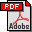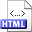Selection from read-only memory with limited workspace Authors: Amr Elmasry, Daniel Dahl Juhl, Jyrki Katajainen, and Srinivasa Rao Satti Published in: Theoretical Computer Science 554 (2014), 64-73 Full text:PDF (336 kB) DOI: 10.1016/j.tcs.2014.06.012 Copyright: © Elsevier B.V. Abstract: Given an unordered array of N elements drawn from a totally ordered set and an integer k in the range from 1 to N, in the classic selection problem the task is to find the k-th smallest element in the array. We study the complexity of this problem in the space-restricted random-access model: The input array is stored on read-only memory, and the algorithm has access to a limited amount of workspace. We prove that the linear-time prune-and-search algorithm—presented in most textbooks on algorithms—can be modified to use Θ(N) bits instead of Θ(N) words of extra space. Prior to our work, the best known algorithm by Frederickson could perform the task with Θ(N) bits of extra space in O(N lg* N) time. Our result separates the space-restricted random-access model and the multi-pass streaming model, since we can surpass the Ω(N lg* N) lower bound known for the latter model. We also generalize our algorithm for the case when the size of the workspace is O(S) bits, where lg3 N ≤ S ≤ N. The running time of our generalized algorithm is O(N lg* (N / S) + N (lg N) / lg S), slightly improving over the O(N lg* (N (lg N) / S) + N (lg N) / lg S) bound of Frederickson’s algorithm. To obtain the improvements mentioned above, we developed a new data structure, called the wavelet stack, that we use for repeated pruning. We expect the wavelet stack to be a useful tool in other applications as well. Related:HTML (Conference paper)HTML (Extended version)HTML (Errata) BibLATEX: ```@article{EJKS2014J, author = {Amr Elmasry and Daniel Dahl Juhl and Jyrki Katajainen and Srinivasa Rao Satti}, title = {Selection from read-only memory with limited workspace}, journaltitle = {Theoretical Computer Science}, volume = {554}, year = {2014}, pages = {64--73}, } ```